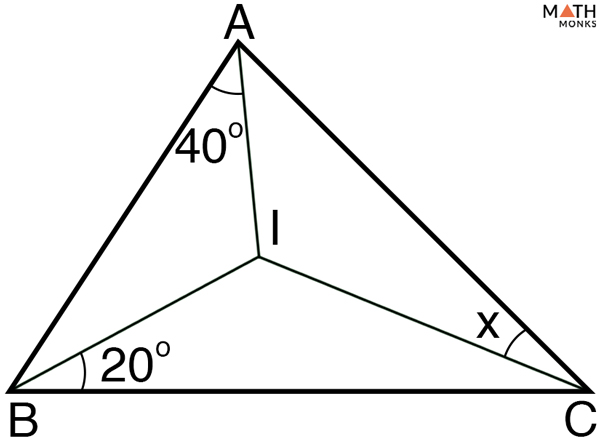# Incenter of a Triangle

## What is the Incenter of a Triangle

The incenter of a triangle is the point where the three interior angle bisectors intersect. The three angle bisectors are always concurrent and always meet in the triangle’s interior. The incenter is thus one of the triangle’s points of concurrency along with the orthocenter, circumcenter, and centroid. It is typically represented by the letter ‘I’.

When a circle is inscribed in a triangle such that the circle touches each side of the triangle, the center of the circle is also called the incenter.

Shown below is a ΔABC with an inscribed circle.

## Properties

1. Found inside a triangle; in ΔABC, ‘I’ is the centroid
2. It is the intersection point of three angle bisectors; in ΔABC, AQ, BR, and CP are the three angle bisectors that meet at the triangle incenter ‘I’
3. It is the center of the triangle’s incircle

Using the properties of incenter, let us solve an example.Find the value of x. Given that ‘I’ is the incenter of the ΔABC.

Solution:

Given: I is the incenter of the ΔABC
Thus, AI, BI, and CI are the angle bisectors.
From, angle sum theorem, we know,
∠BAI + ∠CBI + ∠ACI = 180°
Now, we can write,
∠BAI + ∠CBI + ∠ACI = 180°/2 (since, ∠BAI, ∠CBI, and ∠ACI are angle bisectors of ∠A, ∠B, and ∠C)
40° + 20° + x = 90°
x = 90° – (40° + 20°)
x = 30°

## Formula

### How to Find the Coordinates of the Incenter of a Triangle

The easiest way to find the incenter is by determining the inradius or the radius of the incircle. The inradius is denoted by the letter ‘r’. Once the inradius is known, each side length can be determined by the length of the inradius, and the intersection of the three lines will be the incenter. This is possible using coordinate geometry.

Although, the most widely used procedure is through the use of the Cartesian coordinate system described below:

Let ABC be a triangle whose three vertices are (x1, y1), (x2, y2), (x3, y3) and AD, BE, and CF are the internal bisectors of ∠A, ∠B, and ∠C.

Again, if a, b, and c is the side lengths opposite vertex A, B and C respectively, such that AB = c, BC = a, and CA = b, then, the formula to find the centroid of the triangle is given below:

Let us solve an example to understand the concept better.

A triangle has vertices at A = (0, 0), B = (14, 0), and C = (5, 12). What are the coordinates of the incenter?

Solution:

Let the vertices be:
A = (0, 0) =(x1, y1)
B = (14, 0) =(x2, y2)
C = (5, 12)=(x3, y3)
Here, we will use the distance formula to find the side lengths a, b and c, which is given below:
Distance (d) = √(x2 – x1)2 + (y2 – y1)2
Now,
BC = a = √(x2 – x1)2 + (y2 – y1)2, herex1 = 0, x2 = 14, y1 = 0, y2 = 0
a = √(14 – 5)2 + ( 12 – 0)2
a = √81 + 144
a = 15
AC = b = √(x2 – x1)2 + (y2 – y1)2, herex1 = 0, x2 = 5, y1 = 0, y2 = 12
b = √(5 – 0)2 + (12 – 0)2
b = √25 + 144
b = 13
AB = c = √(x2 – x1)2 + (y2 – y1)2, herex1 = 0, x2 = 14, y1 = 0, y2 = 0
c = √(14 – 0)2 + (0 – 0)2
c = 14
Now, as we know,
Incenter (I) of a triangle = (ax1 + bx2 + cx3/a + b + c, ay1 + by2 + cy3/a + b + c), here vertices A = (0, 0), B = (14, 0), and C = (5, 12)and side lengths BC = 15, AC = 13, & AB = 14
I = (15 × 0 + 13 × 14 + 14 × 5/13 + 14 + 15, 15 × 0 + 13 × 0 + 14 × 12/13 + 14 + 15)
I = (15 + 182 + 70/42, 0 + 0 + 168/42)
I = (6, 4)
Thus, the incenter of the triangle lies at (6, 4)
For a triangle with semiperimeter (s), where s = a + b + c/2 (a, b and c are the side lengths) and inradius (r), the area of the triangle is determined using the formula given below:
Area (A) = s × r

Find the area of the triangle with sides 3 cm, 4 cm, and 5 cm and inradius 2 cm

Solution:

As we know,
Semiperimeter (s) = a + b + c/2, here a = 3 cm, b = 4 cm, and c = 5 cm
= (3 + 4 + 5)/2 = 6 cm
Now,
Area (A) = = s × r, here s = 6 cm, r = 2 cm
= 6 × 2 = 12 cm2

## Constructing Incenter of a Triangle

To construct the incenter of a given triangle one needs to follow the following steps given below.

Steps: